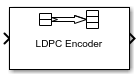# LDPC Encoder

Encode binary low-density parity-check (LDPC) code

•Libraries:
Communications Toolbox / Error Detection and Correction / Block

## Description

The LDPC Encoder block applies LDPC coding to a binary input message. LDPC codes are linear error control codes with sparse parity-check matrices and long block lengths that can attain performance near the Shannon limit.

The input and output are discrete-time signals. The ratio of the output sample time to the input sample time is K/N, where:

• N is the length of the received signal and must be in the range (0, 231).

• K is the length of the uncoded message and must be less than N.

## Ports

### Input

expand all

Input message, specified as a K-by-1 column vector containing binary-valued elements. K is the length of the uncoded message.

Data Types: `double` | `Boolean`

### Output

expand all

LDPC codeword, returned as an N-by-1 column vector. N is the number of bits in the LDPC codeword. The output signal inherits its data type from the input signal. The LDPC codeword output is a solution to the parity-check equation. The input message comprises the first K bits of the LDPC codeword output, and the parity check comprises the remaining (NK) bits.

Data Types: `double` | `Boolean`

## Parameters

expand all

Parity-check matrix, specified as a sparse (NK)-by-N binary-valued matrix. N is the length of the output LDPC codeword and must be in the range (0, 231). K is the length of the uncoded message and must be less than N. The last (NK) columns in the parity-check matrix must be an invertible matrix in the Galois field of order 2, `gf`(2).

You can also specify the parity-check matrix as a two-column nonsparse index matrix, `I`, that defines the row and column indices of the `1`s in the parity-check matrix such that `sparse(I(:,1),I(:,2),1)`.

This parameter accepts numeric data types. When you set this parameter to a sparse binary matrix, this parameter also accepts the `Boolean` data type.

The default value uses the `dvbs2ldpc` function to configure a sparse parity-check matrix for half-rate LDPC coding, as specified in the DVB-S.2 standard.

Note

• When the last (NK) columns of the parity-check matrix form a triangular matrix, forward or backward substitution is performed to solve the parity-check equation.

• When the last (NK) columns of the parity-check matrix do not form a triangular matrix, a matrix inversion is performed to solve the parity-check equation. If a large matrix needs to be inverted, initializations or updates take more time.

Example: `dvbs2ldpc(R,'indices')` configures the index matrix for the DVB-S.2 standard, where `R` is the code rate, and `'indices'` specifies the output format of `dvbs2ldpc` as a two-column double-precision matrix that defines the row and column indices of the `1`s in the parity-check matrix.

## Block Characteristics

 Data Types `Boolean` | `double` | `integer` | `single` Multidimensional Signals `no` Variable-Size Signals `no`

## Version History

Introduced in R2007a